Test: FIR System Structures - 1

# Test: FIR System Structures - 1

Test Description

## 15 Questions MCQ Test Signals and Systems | Test: FIR System Structures - 1

Test: FIR System Structures - 1 for Electrical Engineering (EE) 2023 is part of Signals and Systems preparation. The Test: FIR System Structures - 1 questions and answers have been prepared according to the Electrical Engineering (EE) exam syllabus.The Test: FIR System Structures - 1 MCQs are made for Electrical Engineering (EE) 2023 Exam. Find important definitions, questions, notes, meanings, examples, exercises, MCQs and online tests for Test: FIR System Structures - 1 below.
Solutions of Test: FIR System Structures - 1 questions in English are available as part of our Signals and Systems for Electrical Engineering (EE) & Test: FIR System Structures - 1 solutions in Hindi for Signals and Systems course. Download more important topics, notes, lectures and mock test series for Electrical Engineering (EE) Exam by signing up for free. Attempt Test: FIR System Structures - 1 | 15 questions in 10 minutes | Mock test for Electrical Engineering (EE) preparation | Free important questions MCQ to study Signals and Systems for Electrical Engineering (EE) Exam | Download free PDF with solutions
 1 Crore+ students have signed up on EduRev. Have you?
Test: FIR System Structures - 1 - Question 1

### In general, an FIR system is described by the difference equation: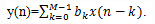Detailed Solution for Test: FIR System Structures - 1 - Question 1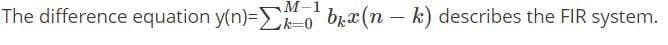Test: FIR System Structures - 1 - Question 2

### Which of the following is an method for implementing an FIR system?

Detailed Solution for Test: FIR System Structures - 1 - Question 2
• There are several structures for implementing an FIR system, beginning with the simplest structure, called the direct form.
• There are several other methods like cascade form realization, frequency sampling realization and lattice realization which are used for implementing and FIR system.
Test: FIR System Structures - 1 - Question 3

### The direct form realization is often called a transversal or tapped-delay-line filter.

Detailed Solution for Test: FIR System Structures - 1 - Question 3

The structure of the direct form realization, resembles a tapped delay line or a transversal system.

Test: FIR System Structures - 1 - Question 4

What is the general system function of an FIR system?

Detailed Solution for Test: FIR System Structures - 1 - Question 4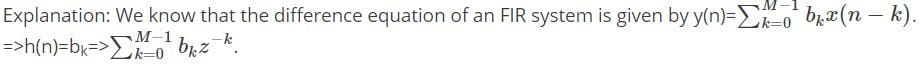Test: FIR System Structures - 1 - Question 5

How many memory locations are used for storage of the output point of a sequence of length M in direct form realization?

Detailed Solution for Test: FIR System Structures - 1 - Question 5
• The direct form realization follows immediately from the non-recursive difference equation given by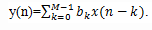• We observe that this structure requires M-1 memory locations for storing the M-1 previous inputs.
Test: FIR System Structures - 1 - Question 6

What is the condition of M, if the structure according to the direct form is as follows?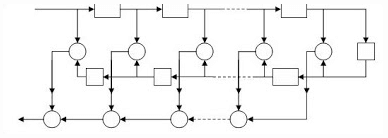Detailed Solution for Test: FIR System Structures - 1 - Question 6
• When the FIR system has linear phase, the unit sample response of the system satisfies either the symmetry or asymmetry condition, h(n)= ±h(M-1-n)
• For such a system the number of multiplications is reduced from M to M/2 for M even and to (M-1)/2 for M odd. Thus for the structure given in the question, M is odd.
Test: FIR System Structures - 1 - Question 7

By combining two pairs of poles to form a fourth order filter section, by what factor we have reduced the number of multiplications?

Detailed Solution for Test: FIR System Structures - 1 - Question 7
• We have to do 3 multiplications for every second order equation.
• So, we have to do 6 multiplications if we combine two second order equations and we have to perform 3 multiplications by directly calculating the fourth order equation.
• Thus the number of multiplications are reduced by a factor of 50%.
Test: FIR System Structures - 1 - Question 8

The desired frequency response is specified at a set of equally spaced frequencies defined by the equation:

Detailed Solution for Test: FIR System Structures - 1 - Question 8
• To derive the frequency sampling structure, we specify the desired frequency response at a set of equally spaced frequencies, namely:
• ωk=2π / M(k+α) ,k=0,1…(M-1)/2 for M odd
• k=0,1….(M/2)-1 for M even α = 0 or 0.5.
Test: FIR System Structures - 1 - Question 9

The realization of FIR filter by frequency sampling realization can be viewed as cascade of how many filters?

Detailed Solution for Test: FIR System Structures - 1 - Question 9
• In frequency sampling realization, the system function H(z) is characterized by the set of frequency samples {H(k+ α)} instead of {h(n)}.
• We view this FIR filter realization as a cascade of two filters. One is an all-zero or a comb filter and the other consists of parallel bank of single pole filters with resonant frequencies.
Test: FIR System Structures - 1 - Question 10

What is the system function of all-zero filter or comb filter?

Detailed Solution for Test: FIR System Structures - 1 - Question 10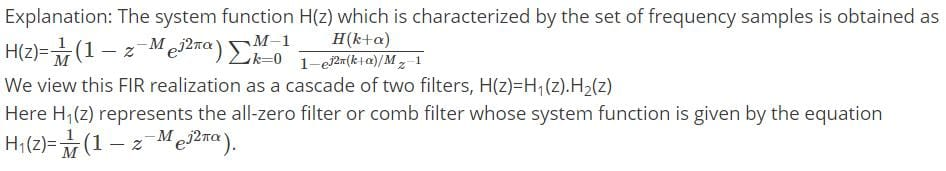Test: FIR System Structures - 1 - Question 11

The zeros of the system function of comb filter are located:

Detailed Solution for Test: FIR System Structures - 1 - Question 11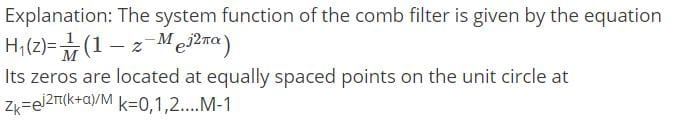Test: FIR System Structures - 1 - Question 12

What is the system function of the second filter other than comb filter in the realization of FIR filter?

Detailed Solution for Test: FIR System Structures - 1 - Question 12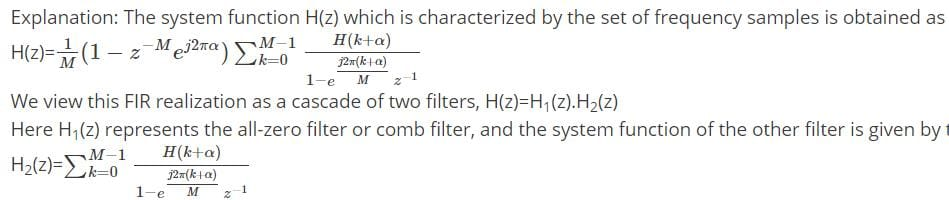Test: FIR System Structures - 1 - Question 13

Where does the poles of the system function of the second filter locate?

Detailed Solution for Test: FIR System Structures - 1 - Question 13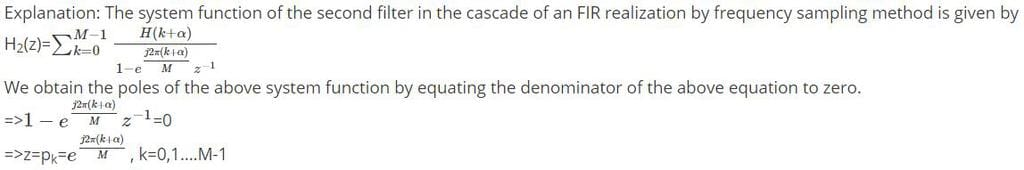Test: FIR System Structures - 1 - Question 14

When the desired frequency response characteristic of the FIR filter is narrowband, most of the gain parameters {H(k+α)} are zero.

Detailed Solution for Test: FIR System Structures - 1 - Question 14

Explanation: When the desired frequency response characteristic of the FIR filter is narrowband, most of the gain parameters {H(k+α)} are zero. Consequently, the corresponding resonant filters can be eliminated and only the filters with nonzero gains need be retained.

Test: FIR System Structures - 1 - Question 15

Which of the following filters have a cascade realization as shown below?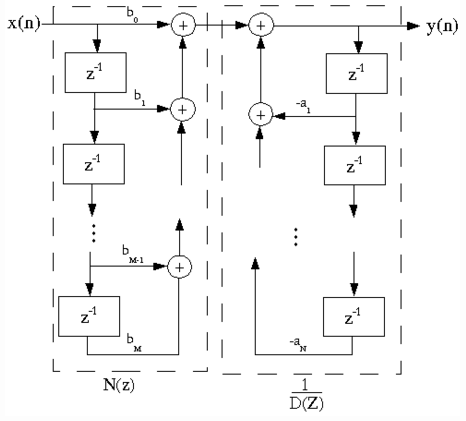Detailed Solution for Test: FIR System Structures - 1 - Question 15

Explanation: The system function of the FIR filter according to the frequency sampling realization is given by the equation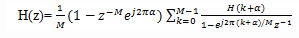The above system function can be represented in the cascade form as shown in the above block diagram.

## Signals and Systems

32 videos|76 docs|63 tests
 Use Code STAYHOME200 and get INR 200 additional OFF Use Coupon Code
Information about Test: FIR System Structures - 1 Page
In this test you can find the Exam questions for Test: FIR System Structures - 1 solved & explained in the simplest way possible. Besides giving Questions and answers for Test: FIR System Structures - 1 , EduRev gives you an ample number of Online tests for practice

## Signals and Systems

32 videos|76 docs|63 tests